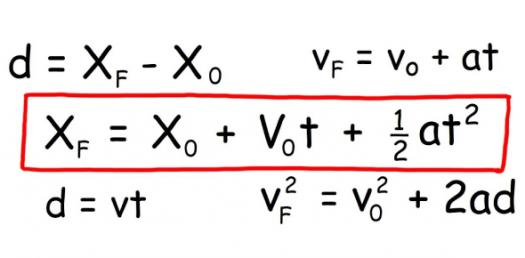# Kinematics: Trivia Questions On Speed,Velocity, And Acceleration! Quiz

5 Questions | Total Attempts: 144Settings.

• 1.
A car moves with a constant speed through 150 m in 5 s.What is its speed?
• A.

30 m/s

• B.

0.033 m/s

• C.

750 m/s

• D.

0 m/s

• 2.
A car travels for 1200 km in 20 hours. The details of the journey are shown below.DurationDistance Covered0 - 6 hours400 km6 - 8 hours0 km(at rest)8 - 16 hours600 km16 - 20 hours200 kmCalculate the average speed of the journey?
• A.

54.5 km/h

• B.

66.67 km/h

• C.

60 km/h

• D.

63.89 km/h

• 3.
An aeroplane moves with a constant speed of 120 m/s.Find the distance travelled in 1 minute?
• A.

120 m

• B.

2 m

• C.

7200 m

• D.

0.5 m

• 4.
Hanafi runs at a constant speed of 3.2 m/s.What distance will he cover in 2 minutes?
• A.

384 m

• B.

6.4 m

• C.

192 m

• D.

1.6 m

• 5.
A bus is parked in the car park. It is not moving.What is its speed?
• A.

0 m/s

• B.

10 m/s

• C.

1 m/s

• D.

5 m/s

Related Topics